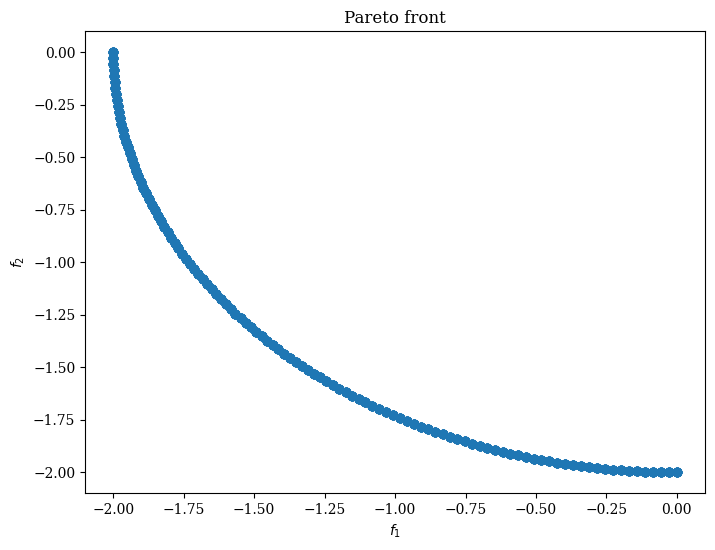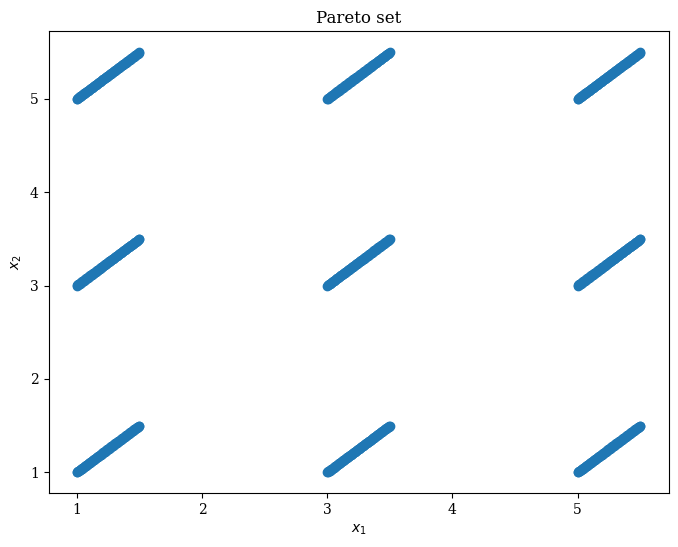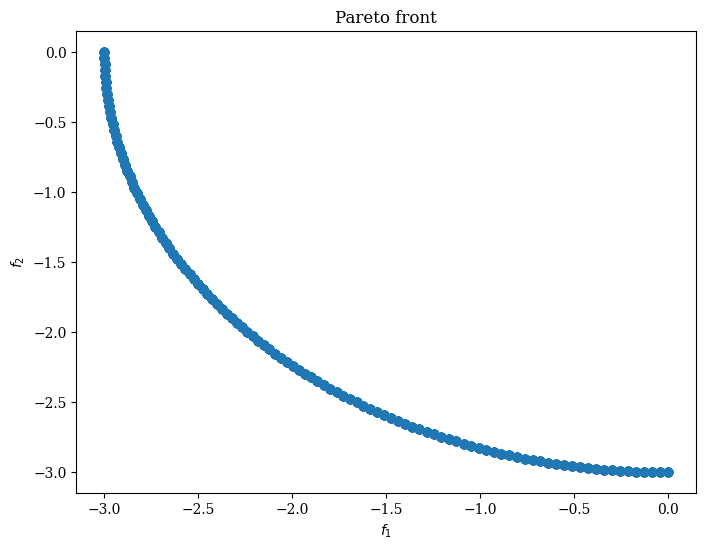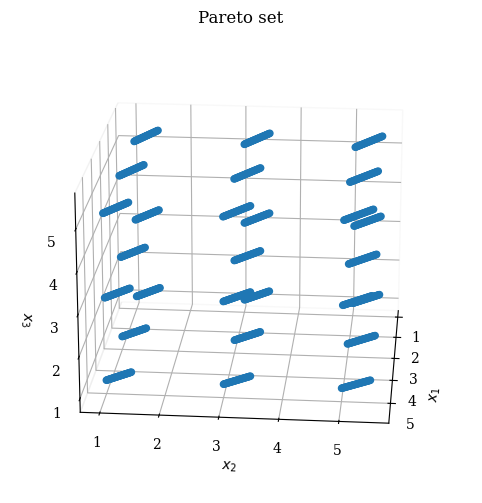Version: 0.5.0

# Omni-test¶

The Omni-test problem is a multi-modal multi-objective optimization problem proposed by Deb in . It has two objective functions. Suppose that the dimension of the decision space is $$D$$, then it has $$3^D$$ Pareto subsets in the decision space corresponding to the same Pareto front.

## 2-dimensional case¶

### Pareto front¶

:

from pymoo.problems.multi.omnitest import OmniTest
from pymoo.visualization.scatter import Scatter

problem = OmniTest(n_var=2)
pf = problem.pareto_front(1000)

:

<pymoo.visualization.scatter.Scatter at 0x7f5561b4f9a0>### Pareto set¶

:

ps = problem.pareto_set(1000)
Scatter(title="Pareto set", labels=["$x_1$", "$x_2$"]).add(ps).show()

:

<pymoo.visualization.scatter.Scatter at 0x7f5561b4fb80>## 3-dimensional case¶

### Pareto front¶

:

problem = OmniTest(n_var=3)
pf = problem.pareto_front(3000)

:

<pymoo.visualization.scatter.Scatter at 0x7f5552915670>### Pareto set¶

:

import matplotlib.pyplot as plt

ps = problem.pareto_set(1000)
sc = Scatter(title="Pareto set", labels=["$x_1$", "$x_2$", "$x_3$"])